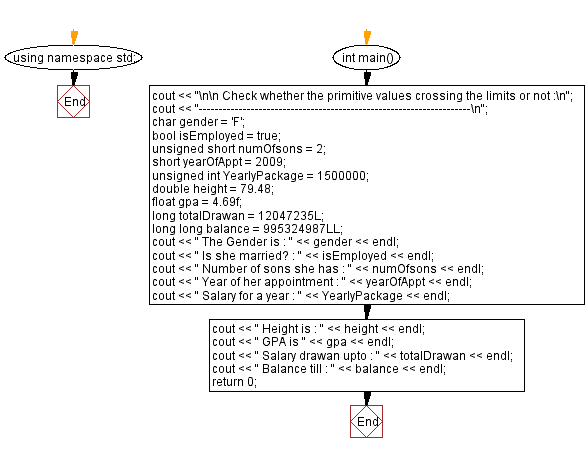﻿ C++ : Check the primitive values crossing the limits or not

# C++ Exercises: Check whether the primitive values crossing the limits or not

## C++ Basic: Exercise-6 with Solution

Write a C++ program that checks whether primitive values cross the limit.

Sample Solution:

C++ Code :

``````#include <iostream>
using namespace std;

int main()
{
cout << "\n\n Check whether the primitive values crossing the limits or not :\n";
cout << "--------------------------------------------------------------------\n";
char gender = 'F';             // char is single-quoted
bool isEmployed = true;         // true(non-zero) or false(0)
unsigned short numOfsons = 2; // [0, 255]
short yearOfAppt = 2009;      // [-32767, 32768]
unsigned int YearlyPackage = 1500000;   // [0, 4294967295]
double height = 79.48;       // With fractional part
float gpa = 4.69f;           // Need suffix 'f' for float
long totalDrawan = 12047235L;     // Suffix 'L' for long
long long balance = 995324987LL;  // Need suffix 'LL' for long long int

cout << " The Gender is : " << gender << endl;
cout << " Is she married? : " << isEmployed << endl;
cout << " Number of sons she has : " << numOfsons << endl;
cout << " Year of her appointment : " << yearOfAppt << endl;
cout << " Salary for a year : " << YearlyPackage << endl;
cout << " Height is : " << height << endl;
cout << " GPA is " << gpa << endl;
cout << " Salary drawn upto : " << totalDrawan << endl;
cout << " Balance till : " << balance << endl;
return 0;
}
``````

Sample Output:

``` Check whether the primitive values crossing the limits or not :
--------------------------------------------------------------------
The Gender is : F
Is she married? : 1
Number of sons she has : 2
Year of her appointment : 2009
Salary for a year : 1500000
Height is : 79.48
GPA is 4.69
Salary drawn upto : 12047235
Balance till : 995324987
```

Flowchart:C++ Code Editor:

What is the difficulty level of this exercise?

﻿

## C++ Programming: Tips of the Day

Why is there no std::stou?

The most pat answer would be that the C library has no corresponding "strtou", and the C++11 string functions are all just thinly veiled wrappers around the C library functions: The std::sto* functions mirror strto*, and the std::to_string functions use sprintf.

Ref: https://bit.ly/3wtz2qA

We are closing our Disqus commenting system for some maintenanace issues. You may write to us at reach[at]yahoo[dot]com or visit us at Facebook